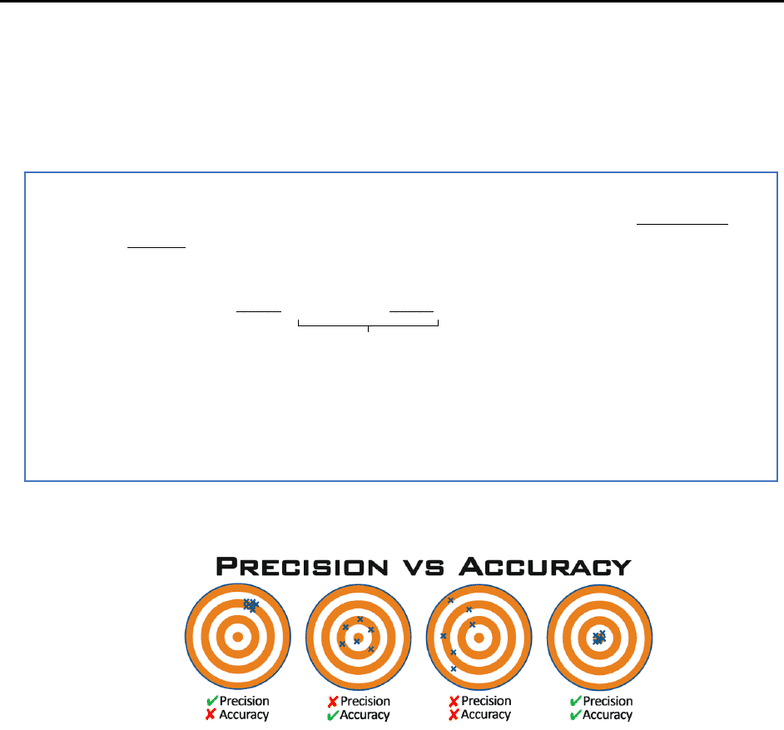# CHM135H1 Study Guide - Midterm Guide: Unified Atomic Mass Unit, Benzocaine, Calcium Carbide

267 views20 pagesUniversity of Toronto (St. George)
CHM139 - Chemistry: Physical Principles
Professor: Kristine Quinlan
Midterm 1
Exam Guide
Topics Included:
1. Review of Fundamental Concepts
2. Quantum Mechanics
3. States of Matter: Solids
4. States of Matter: Liquids
5. States of Matter: Gases
6. Solutions
OneClass
Unlock document

This preview shows pages 1-3 of the document.
Unlock all 20 pages and 3 million more documents.Unit 1: Chapters 1-5
Part 1 Review of Fundamental Concepts
1.1 Measurements and Observations
Measurements contain number and scale
Units are very important when plugging values into equations and formulas as you need to
make sure that you are using standard units
Accuracy and precision are two different concepts that you should not confuse with one another
Accuracy how close the measurement is to the true value
Precision how close repeated measurements are to each other
Keep in mind that all measurements are subject to error so use significant figures (sig figs)
because they reflect the precision of the measurement
o Significant figures are the total number of digits in the measurement
Matter is anything that has both mass and volume
Mixtures are two or more substances mixed without chemically altering components and there
are two types:
o Heterogeneous mixture not uniform
o Homogeneous mixture same throughout
Important notes:
Units can be a hint to getting the right answer to a problem through dimensional
analysis (unit conversion) because if you get the right units for the answer, it shows
that you have plugged in the right values (however it does not mean that the answer
is correct!)
Measurement unit A × (x unit B ÷ y unit A)
conversion factor
Ex: At 20°C, the density of water is 0.9978g/ml. What volume will be occupied by
1.75kg of water at this temperature?
Solution: 1.75kg × (1000g ÷ 1kg) × (1mL ÷ 0.9978g/mL) = 1.75×103mL
OneClass
Unlock document

This preview shows pages 1-3 of the document.
Unlock all 20 pages and 3 million more documents.Pure Substances have definite/constant composition such as elements and chemical compounds
1.2 The Atom
Dalton’s Atomic Theory sates that:
o Elements are made of tiny particles called atoms
o Atoms of each element are identical and different from atoms of other elements
o Chemical compounds are made of atoms combined in small whole number ratios
o In chemical reactions, the atoms merely reorganize (the atoms themselves don’t
change)
Elements are fundamental substances that can’t be chemically changed to broken down into
something simpler and are organized in the periodic table
An atom is neutral overall with a positively charged central nucleus (containing positively
charged protons and neutral neutrons) that is surrounded by negatively charged electrons
Atoms of each element have the same number of protons (and electrons), however it is possible
for the same element to have a different number of neutrons and these are called isotopes
o Different isotopes of an element have different masses
Atoms are tiny and the defined unit called atomic mass unit (amu) 1amu = 1.66×10-24g
Ex: 12C 12amu 98.89% and 13C 13.0034amu 1.11% What is the elemental average mass?
Solution: 12amu(0.9889) + 13.0034amu(0.011) = 12.01amu
Molecules are created when atoms interact at short distances to form chemical bonds
o Covalent Bonding atoms share electrons to form bond
o Ionic Bonding electrons are transferred and the resulting ions are attracted to each
other
1.3 Stoichiometry
Atoms are very tiny so it’s more convenient to consider a bunch together such as a mole
o 1 mol = 6.022×1023 units of something and this number is known as Avogadro’s number
(NA)
Molar mass (found on periodic table) gives the mass of 1mol of atoms
The empirical formula is the chemical formula expressed using the smallest possible whole
numbers
o Ex: HO for H2O2 gives ratios of atoms, not actual numbers of atoms
Important notes:
Notation
o n amount of substance (mol)
o MM molar mass (g/mol)
o m mass (g)
Equation:
o n = m ÷ MM
OneClass
Unlock document

This preview shows pages 1-3 of the document.
Unlock all 20 pages and 3 million more documents.

## Document Summary

Topics included: review of fundamental concepts, quantum mechanics, states of matter: solids, states of matter: liquids, states of matter: gases, solutions. Units are very important when plugging values into equations and formulas as you need to make sure that you are using standard units. Measurement unit a (x unit b y unit a) conversion factor. Ex: at 20 c, the density of water is 0. 9978g/ml. Solution: 1. 75kg (1000g 1kg) (1ml 0. 9978g/ml) = 1. 75 103ml. Accuracy and precision are two different concepts that you should not confuse with one another. Accuracy how close the measurement is to the true value. Precision how close repeated measurements are to each other. Keep in mind that all measurements are subject to error so use significant figures (sig figs) because they reflect the precision of the measurement. Significant figures are the total number of digits in the measurement. Matter is anything that has both mass and volume.